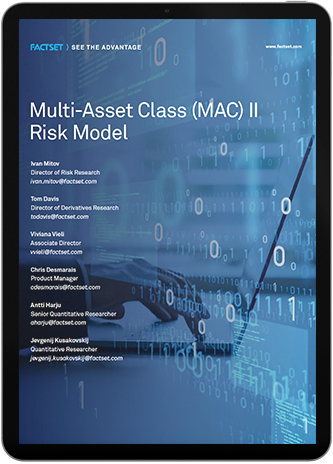# White Paper Multi-Asset Class Risk Model II

## White Paper: Multi-Asset Class Risk Model IIMulti-asset class (MAC) risk factor models present unique challenges due to the complexity of calculating risks across different asset types. However, they are becoming absolutely necessary now as more investment managers are operating on global, multi-asset-type markets. Historically, the risks of such portfolios have been estimated by independently modeling risks of holdings of each asset type, like equities or fixed income, and then aggregating those risks into a MAC portfolio risk. This can result in significant errors in estimated risk because this methodology does not consider correlations between different asset classes. A MAC risk factor model that is based on a set of factors that capture systematic shifts in all markets (e.g., equities, fixed income, commodities) remedies this deficiency and allows the portfolio manager to accurately estimate portfolio risk and its components stemming from different asset type markets.

Download the Multi-Asset Class Risk Model II  white paper to uncover the structure of the MAC factor risk framework and the types of factors used in its models. Learn about the estimation techniques used to compute risk factors and the corresponding security returns.